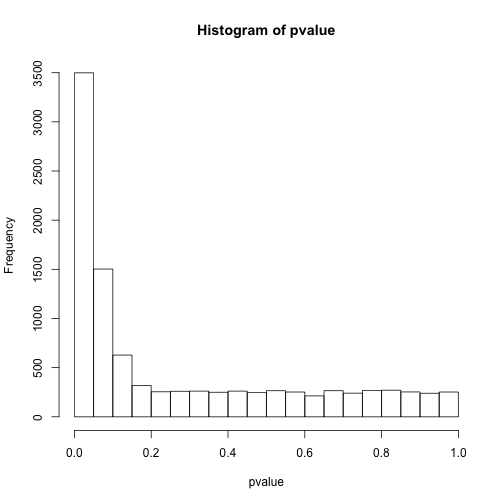# 高通量数据的多重检验问题

# 随机数的10000次比较

set.seed(42)

pvalue<-NULL

for(i in1:10000){a<-rnorm(10);b<-rnorm(10);c<-t.test(a,b);pvalue[i]<-c$p.value} # 看下p值分布 hist(pvalue)# 小于0.05的个数sum(pvalue<0.05) ##  477 # 小于0.0001的个数sum(pvalue<0.0001) ##  0 这样我们会看到进行了整体的控制之后，确实是找不到有差异的了，但假如里面本来就有有差异的呢？ set.seed(42) pvalue<-NULL for(i in1:10000){a<-rnorm(10,1);b<-a+1;c<-t.test(a,b);pvalue[i]<-c$p.value}

# 看下p值分布

hist(pvalue)# 小于0.05的个数sum(pvalue<0.05)
##  6559
# 小于0.0001的个数sum(pvalue<0.0001)
##  45

set.seed(42)

pvalue<-NULL

for(i in1:5000){a<-rnorm(10,1);b<-a+1;c<-t.test(a,b);pvalue[i]<-c$p.value} for(i in1:5000){a<-rnorm(10,1);b<-rnorm(10,1);c<-t.test(a,b);pvalue[i+5000]<-c$p.value}

# 看下p值分布

hist(pvalue)# 小于0.05的个数sum(pvalue<0.05)
##  3499
# 小于0.0001的个数sum(pvalue<0.0001)
##  21

Bonferroni 方法

P(至少一个显著)=1-P(无显著差异) = 1-(1-\alpha/n)^n

n<-c(1:10%o%10^(1:2))

p0<-1-(1-0.05)^n

p<-1-(1-0.05/n)^n

# 不进行控制

plot(p0~n,ylim=c(0,1))

# Bonferroni方法控制

points(p~n,pch=19)Sidak 方法

P(至少一个显著)=1−P(无显著差异)=1−(1−α′)n=0.05

# 所有有差异的

R<-sum(pvalue<0.05)

# 假阳性

V<-sum(pvalue[5001:10000]<0.05)

# 错误发现率

Q<-V/RR

##  3499
V
##  225
Q
##  0.06430409

# 所有有差异的

R<-sum(pvalue<0.01)

# 假阳性

V<-sum(pvalue[5001:10000]<0.01)

# 错误发现率

Q<-V/RR

##  999
V
##  34
Q
##  0.03403403

Benjamini-Hochberg方法

plot(pbh~pvalue)points(ph~pvalue,col='red')Storey方法（q值）

$\hat\pi_0 = \frac{\#\{p_i>\lambda\}}{(1-\lambda)m}$

library(qvalue)

q<-qvalue(pvalue)

# Q值

plot(q$qvalues~pvalue,col='blue')如上图所示，q值增大后会最终逼近到0.5，而我们的模拟中空假设的比例就设定就是50%。我们重新模拟一个空假设比例5%的实验： set.seed(42) pvalue<-NULL for(i in1:500){a<-rnorm(10,1);b<-a+1;c<-t.test(a,b);pvalue[i]<-c$p.value}

for(i in1:9500){a<-rnorm(10,1);b<-rnorm(10,1);c<-t.test(a,b);pvalue[i+500]<-c$p.value} pbh<-p.adjust(pvalue,method='BH') ph<-p.adjust(pvalue,method='holm') q<-qvalue(pvalue)plot(pbh~pvalue) # Holm 方法 points(ph~pvalue,col='red') # Q值 points(q$qvalues~pvalue,col='blue')https://wap.sciencenet.cn/blog-430956-1026461.html

## 相关博文

GMT+8, 2021-8-6 12:58